# Integers

Go back to  'Numbers'

 1 What is an Integer? 2 Integer Operations 3 Integers Calculator 4 Challenging Questions on Integers 5 Properties of Integers 6 Thinking out of the Box! 7 Solved Examples on Integers 8 Practice Questions on Integers 9 Important Topics 10 Maths Olympiad Sample Papers 11 Frequently Asked Questions (FAQs)

We at Cuemath believe that Math is a life skill. Our Math Experts focus on the “Why” behind the “What.” Students can explore from a huge range of interactive worksheets, visuals, simulations, practice tests, and more to understand a concept in depth.

Book a FREE trial class today! and experience Cuemath’s LIVE Online Class with your child.

## What is an Integer?

An integer is a number with no decimal part.

The set of integers is $$\{..., -3, -2, -1, 0, 1, 2, 3,...\}$$ and is represented by $$Z$$.

 $$Z= \{... ,-3, -2, -1, 0, 1, 2, 3,...\}$$

The set of integers include:

• natural numbers $$1, 2, 3, ...$$
• the number $$0$$.
• negatives of natural numbers $$-3, -2, -1, ...$$

Some examples of integer numbers are $$-52, -3, 0, 27,$$ etc.

Some examples of non-integers are \begin{align}0.5, \frac{3}{4}, - \sqrt{2} \end{align} etc.

### Integer Number Line

The set of integers can be represented by a number line.

On the number line:

• positive numbers should be on the right side of $$0$$ as they are greater than $$0$$
• negative numbers should be on the left side of $$0$$ as they are lesser than $$0$$

$$0$$ is neither positive nor negative.

The integer number line is shown below and it extends infinitely on both sides.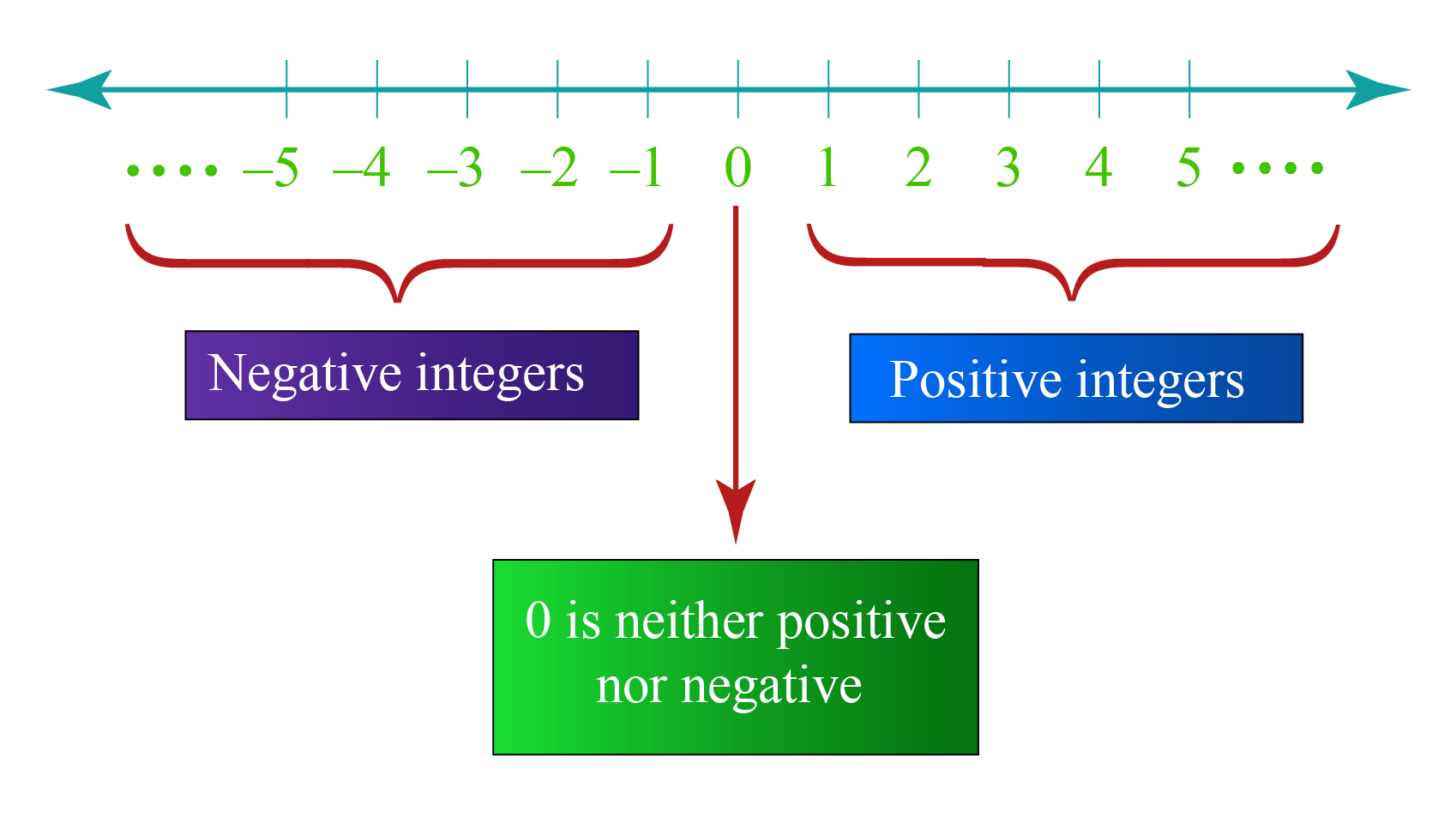## Integer Operations

Integers can be added, subtracted, multiplied or divided.

There are some rules for doing these operations.

Before we learn these methods of integer operations, we need to remember few things.

• If there is no sign in front of a number, it means that it is positive.

For example, $$5$$ means $$+5$$.

• The absolute value of an integer is a positive number. i.e.,
\begin{align}|-2| &=2 \\ |2|& =2\end{align}

While adding two integers, we come across these cases.

• When both integers have the same signs, add their absolute values and give the same sign as that of the given integers to the result.

Example 1:

Calculate the value of $$2+3$$

Solution:

We already know that $$2+3=5$$

Example 2:

Calculate the value of $$-2+(-3)$$

Solution:

Here, the absolute values of $$-2$$ and $$-3$$ are $$2$$ and $$3$$ respectively.

Since both the integers have the same sign, we have to add their absolute values first. i.e., $$2+3=5$$

Since the signs of both of the given integers is "$$-$$", the result also get the same sign, "$$-$$"  $$-2+(-3) = -5$$

• When one integer is positive and the other is negative, we first take the difference of the absolute values of the numbers and then give the original sign of the biggest of these numbers to the result.

Example 1:

Calculate the value of $$2+(-5)$$

Solution:

Here the absolute values of $$2$$ and $$-5$$ are $$2$$ and $$5$$ respectively.

Their difference (larger number - smaller number) is $$5-2=3$$

Now, among $$2$$ and $$5$$, $$5$$ is the bigger number and its original sign is "$$-$$" in the problem.

Hence, the result gets the negative sign, "$$-$$"  $$2+(-5) =-3$$

Example 2:

Calculate the value of $$-2+5$$

Solution:

By solving this example in the same way as above, we can say that:   $$-2+ 5 = 3$$

To show the addition of integers on the number line, the rules are:

• always start from $$0$$
• move to the right side, if the number is positive.
• move to the left side, if the number is negative.

Let's find the value of $$5+(-10)$$ using the number line.

In the given problem, the first number is $$5$$ which is positive.

We start from $$0$$ and move $$5$$ units to the right side.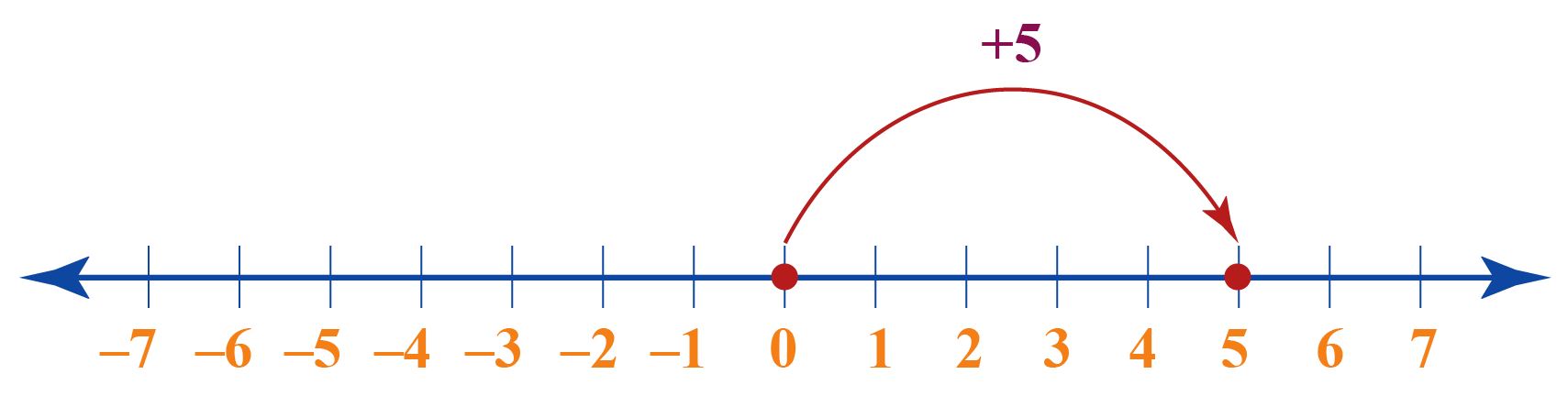The next number in the given problem is $$-10$$ which is negative.

We move (from the fifth unit) $$10$$ units to the left side.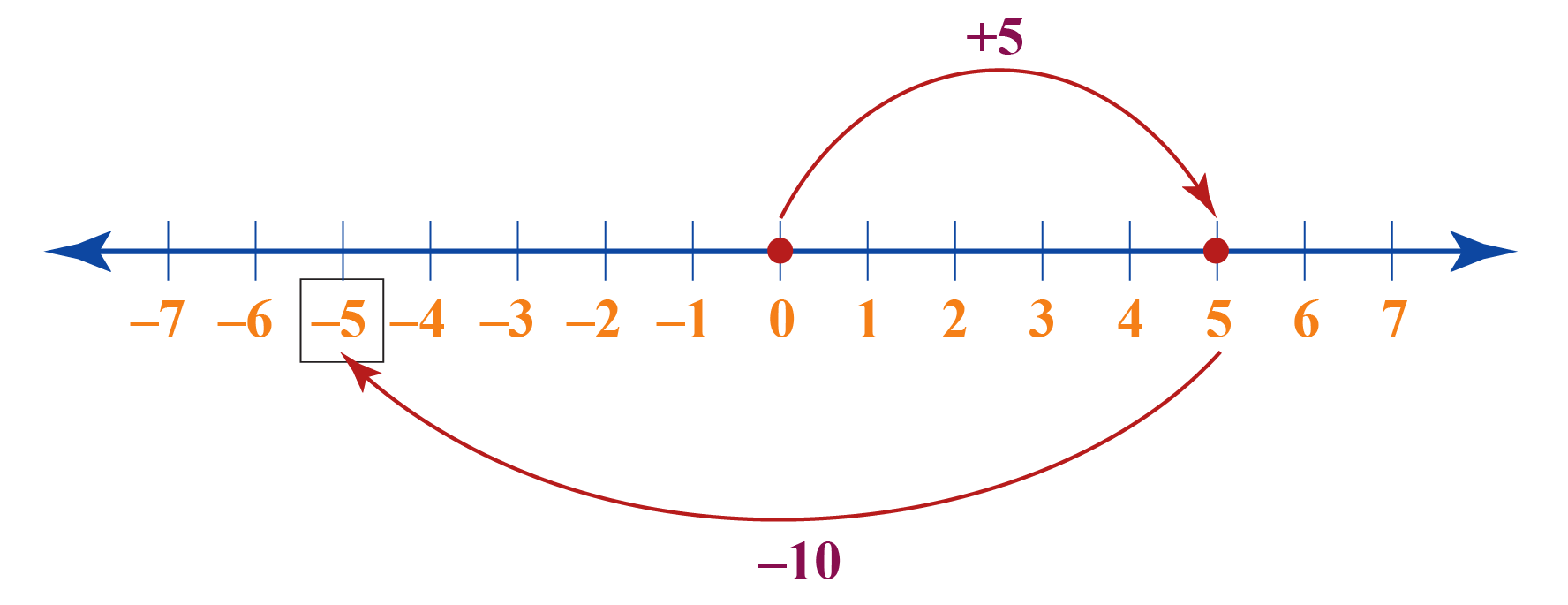The number we have moved to finally is $$-5$$

Thus,

 $$5+(-10)=-5$$

### Subtraction of Integers

This is the method to subtract two integers.

• First, we have to convert it into an addition problem by changing the sign of the subtrahend.

For example, $7 - (10)=7+ (-10)$

• Next, we have to apply the same rules of addition of integers and solve the above problem.

Thus, the above example becomes, $7 - (10)=7+ (-10) =-3$

### Multiplication of Integers

We have to do the following steps to multiply two integers.

Step 1: First, we have to multiply their signs and get the resultant sign.

Step 2: Next, we have to multiply the numbers and add the resultant sign to the answer.

Product of Sign Result Examples
$$+ \times +$$ $$+$$ $$2 \times 3 = 6$$
$$+ \times -$$ $$-$$ $$-2 \times -3 = 6$$
$$- \times +$$ $$-$$ $$-2 \times 3 = -6$$
$$- \times -$$ $$+$$ $$2 \times -3 =-6$$

Next, to show multiplication on the number line, we should consider multiplication as repeated addition.

Example 1:

Using the number line, find the value of

$2 \times -3$

Solution:

We read $$2 \times -3$$ as "$$2$$ times $$-3$$"

We have to represent $$-3$$ on the number line $$2$$ times.

To do so, we will start from $$0$$ and move left by $$3$$ units twice.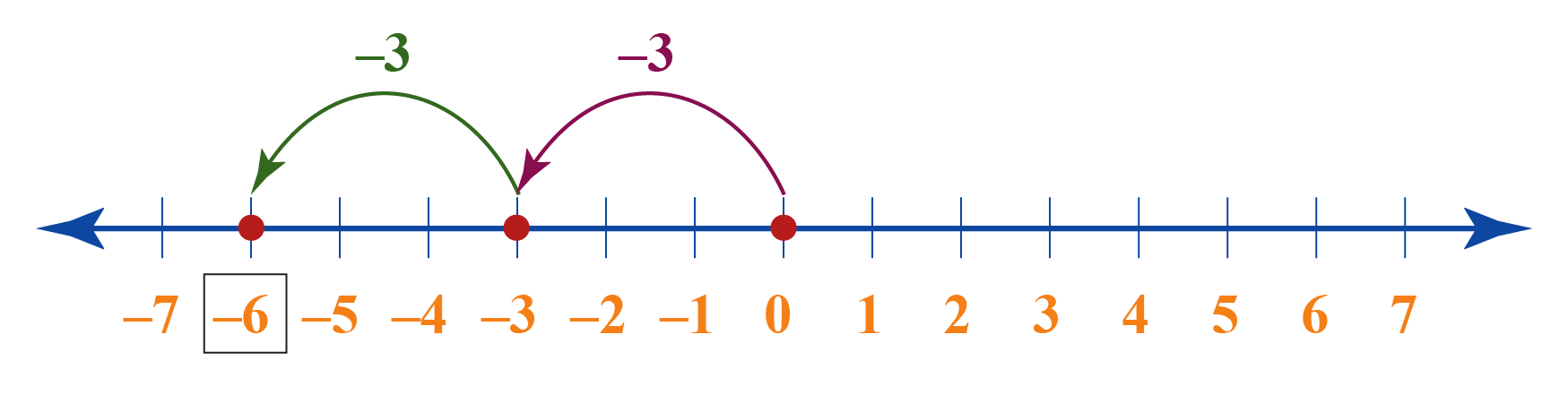Thus,

 $$2 \times -3=-6$$

Example 2:

Using the number line, find the value of

$-2 \times -3$

Solution:

The given problem is similar to the above problem, but $$2$$ is replaced by $$-2$$

Hence, we follow the same number line process as above but in the opposite direction (i.e., to the right side).

The number line will be represented in this way: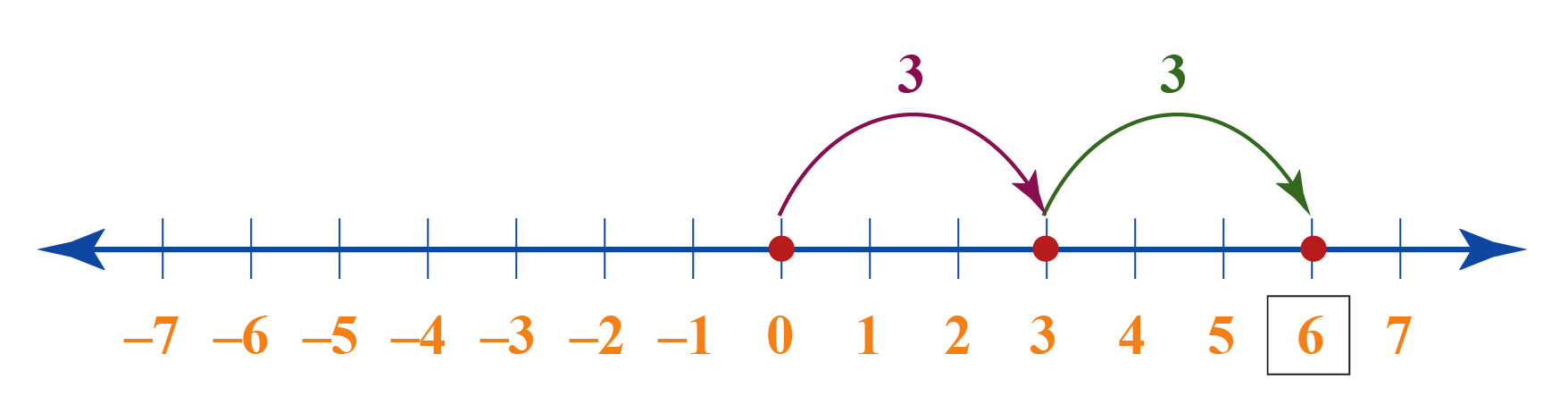Thus,

 $$-2 \times -3=6$$

### Division of Integers

The method to divide two integers is explained below.

Step 1: First, we will divide their signs and get the resultant sign.

Step 2: Next, we will divide the numbers and add the resultant sign to the answer.

Product of Sign Result Examples
$$+ \div +$$ $$+$$ $$12 \div 3 = 4$$
$$+ \div -$$ $$-$$ $$-12 \div -3 = 4$$
$$- \div +$$ $$-$$ $$-12 \div 3 = -4$$
$$- \div -$$ $$+$$ $$12 \div -3 = -4$$

We observe, that the rules are the same as that of multiplication.

## Integers Calculator

Here is the "Integers Calculator".

You can enter any two integers here.

It can show you their sum/difference/product/quotient.Challenging Questions
1. Solve $$300 + (-20) - (-350)$$
2. Solve $$(-2 \times 3) - 8$$
3. Solve $$(-150 \div 3) + 16$$?

CLUEless in Math? Check out how CUEMATH Teachers will explain Integers to your kid using interactive simulations & worksheets so they never have to memorize anything in Math again!

Explore Cuemath Live, Interactive & Personalised Online Classes to make your kid a Math Expert. Book a FREE trial class today!

## Properties of Integers

Let us assume that $$a, b$$ and $$c$$ are any three integers.

We will understand the properties of integers using the following table:

 Closure Property $$a+b$$ is an integer $$Z$$ is closed under addition. $$a \times b$$ is an integer $$Z$$ is closed under multiplication. Associative Property $$a+(b+c) = (a+b)+c$$ $$Z$$ is associative under addition. $$a \times (b \times c) = (a\times b)\times c$$ $$Z$$ is associative under multiplication. Commutative Property $$a+b$$ = $$b+a$$ $$Z$$ is commutative under addition. $$a \times b$$ = $$b \times a$$ $$Z$$ is commutative under multiplication. Additive Identity $$a+0=0+a=a$$ Here $$0$$ is the additive identity. Additive identity exists in $$Z$$. Multiplicative Identity $$a \times 1 = 1 \times a = a$$ Here $$1$$ is the multiplicative identity. Multiplicative identity exists in $$Z$$. Additive Inverse $$a+(-a)= (-a)+a=0$$ Here, $$a$$ and $$-a$$ are the additive inverses of each other. Every element of $$Z$$ has its additive inverse in $$Z$$. Multiplicative Inverse $$a \times \frac{1}{a} = \frac{1}{a} \times a=1$$ Here, $$a$$ and $$\frac{1}{a}$$ are the multiplicative inverses of each other. No element of $$Z$$ has its multiplicative inverse in $$Z$$.Think Tank
1. Is $$Z$$ closed under subtraction and division?
2. Is $$Z$$ associative under subtraction and division?
3. Is $$Z$$ commutative under subtraction and division?

Help your child score higher with Cuemath’s proprietary FREE Diagnostic Test. Get access to detailed reports, customized learning plans, and a FREE counseling session. Attempt the test now.

## Solved Examples

 Example 1

Identify the property used.

$-3 \times -7 = -7 \times -3$

Solution:

The commutative property says:

$a \times b = b \times a$

Therefore,

 The Property used = Commutative Property
 Example 2

A plane is flying at the height of $$3000$$ m above the sea level.

At some point, it is exactly above the submarine floating $$700$$ m below the sea level.

What is the vertical distance between them?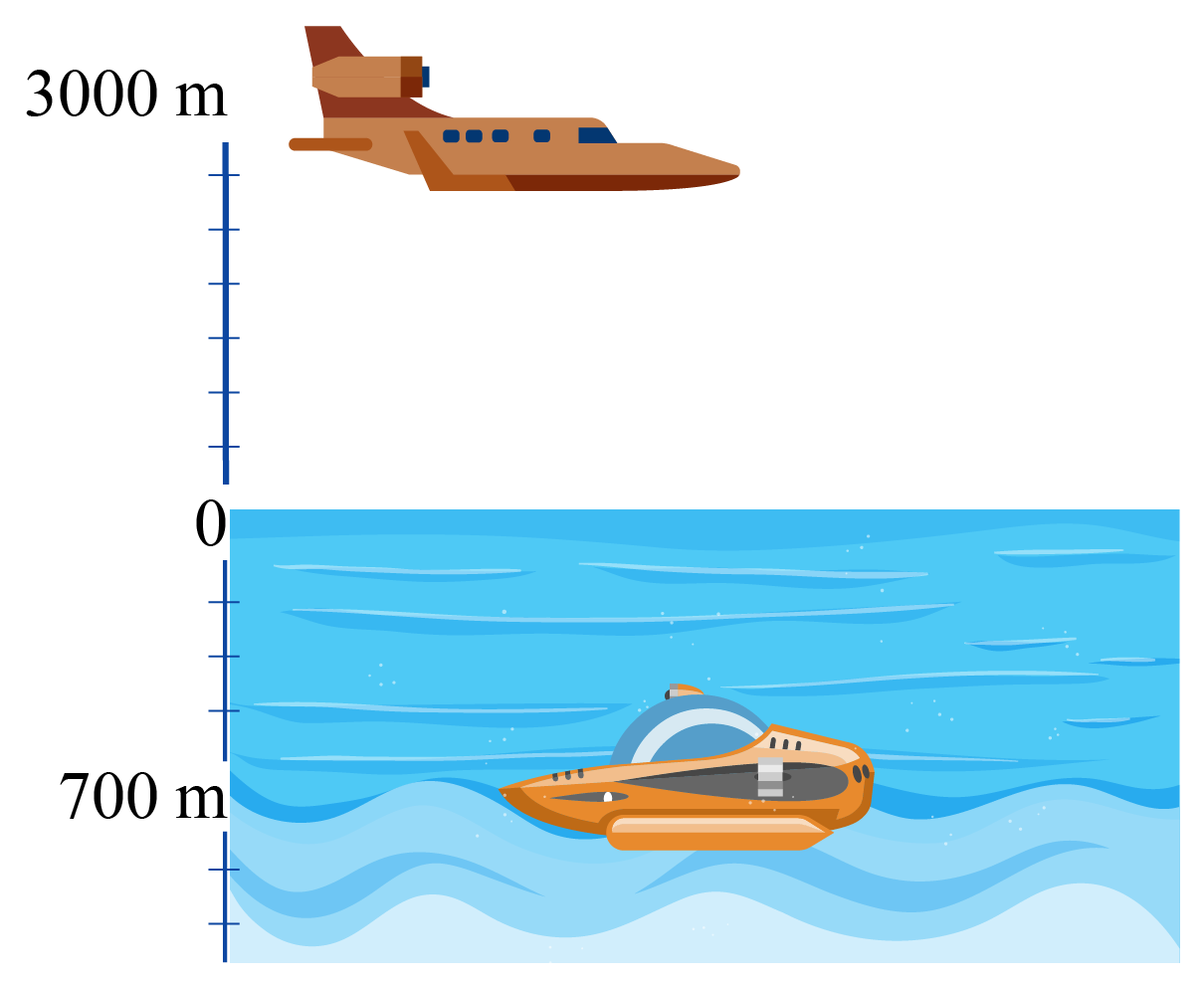Solution:

The height at which the plane is flying = $$3000$$ m

The depth of the submarine = $$-700$$ m (Negative as it is below the sea level)

The vertical distance between them is:

$3000 - (-700) = 3000 + 700 = 3700$

 Vertical Distance = $$3700$$ m
 Example 3

A class test contains $$20$$ questions where each correct answer is awarded $$4$$ marks.

Each incorrect answer is awarded with $$-2$$ marks (negative marks).

If a student answered $$9$$ questions correctly and answered the remaining questions incorrectly, what is his score?

Solution:

Total number of questions = $$20$$

The number of questions answered correctly = $$9$$

Thus, the associated score = $$9 \times 4 = 36$$

The number of questions answered incorrectly = $$20-9=11$$

The associated score will be = $$11 \times -2 = -22$$

Thus, the total score = $$36+(-22) = 14$$

 Total score = $$14$$

## Important Topics

Given below are the list of topics that are closely connected to integers. These topics will also give you a glimpse of how such concepts are covered in Cuemath.

IMO (International Maths Olympiad) is a competitive exam in Mathematics conducted annually for school students. It encourages children to develop their math solving skills from a competition perspective.

## 1. What is an integer in simple terms?

An integer is any number that belongs to the set $$Z= \{...,-3,-2,-1,0,1,2,3,...\}$$

Some integer numbers are $$-3, 0, 31,$$ etc.

Some non-integers are $$3.7, -0.1, \frac{1}{2},$$ etc.

## 2. Is a decimal an integer?

No, a decimal is NOT an integer.

Some integers examples are $$-32, 51, 0$$, etc.

## 3. Is the number 0 an integer?

Yes, $$0$$ is an integer.

$$0$$ is an integer which is neither positive nor negative.

## 4. What are the integers from 1 to 10?

The integers from $$1$$ to $$10$$ are $$1,2,3,4,5,6,7,8,9,10$$.

## 5. What is another word for integer?

"Integer number" is also known as "integral number."

## 6. What are the types of integers?

Integers are of three types. They are:

• positive integers which are natural numbers $$1,2,3,...$$
• zero $$(0)$$.
• negative integers which are negatives of natural numbers $$...,-3,-2,-1$$

## 7. Are negative numbers integers?

Negative numbers without the fraction part are integers.

For example:

$$-2, -3, -31$$ are some integers examples.

$$-2.5, -3.7, - \frac{3}{2}$$ are some non-integers examples.

Arithmetic Integers
grade 10 | Questions Set 2
Arithmetic Integers
Arithmetic Integers
grade 10 | Questions Set 1
Arithmetic Integers
An Introduction to Integers
An Introduction to Integers
Integers-A Consolidation
Integers-A Consolidation
Numbers and Number Systems
grade 9 | Questions Set 2
Numbers and Number Systems
Numbers and Number Systems
Numbers and Number Systems
grade 9 | Questions Set 1
Numbers and Number Systems